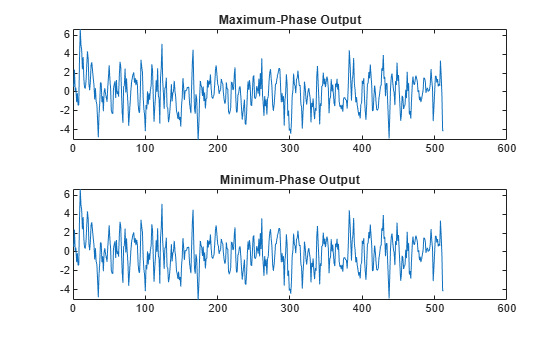# latcfilt

## Syntax

``[f,g] = latcfilt(k,x)``
``[f,g] = latcfilt(k,v,x)``
``[f,g] = latcfilt(k,1,x)``
``[f,g,zf] = latcfilt(___,"ic",zi)``
``[f,g,zf] = latcfilt(___,dim)``

## Description

example

````[f,g] = latcfilt(k,x)` filters input signal `x` with the FIR lattice coefficients specified by `k` and returns the forward lattice filter result `f` and backward filter result `g`.```
````[f,g] = latcfilt(k,v,x)` filters input signal `x` with the IIR lattice coefficients specified by `k` and ladder coefficients specified by `v`. Both `k` and `v` must be vectors, while `x` can be a matrix.```
````[f,g] = latcfilt(k,1,x)` filters input signal `x` with the IIR lattice coefficients specified by `k` and returns the all-pole lattice filter result `f` and the allpass filter result `g`.```
````[f,g,zf] = latcfilt(___,"ic",zi)` specifies the initial conditions of the lattice states `zi` and returns the final conditions of the lattice states `zf`.```
````[f,g,zf] = latcfilt(___,dim)` filters `x` along the dimension `dim`.```

## Examples

collapse all

Generate a signal with 512 samples of white Gaussian noise.

`x = randn(512,1);`

Filter the data with an FIR lattice filter. Specify the reflection coefficients so that the lattice filter is equivalent to a 3rd-order moving average filter.

`[f,g] = latcfilt([1/2 1],x);`

Plot the maximum- and minimum-phase outputs of the lattice filter in separate plots

```subplot(2,1,1) plot(f) title('Maximum-Phase Output') subplot(2,1,2) plot(g) title('Minimum-Phase Output')```## Input Arguments

collapse all

Lattice coefficients, specified as a vector or matrix. If $|k|\le 1$, `f` corresponds to the minimum-phase output, and `g` corresponds to the maximum-phase output.

When filtering data, lattice coefficients can be used to represent

• FIR filters

• All-pole IIR filters

• Allpass IIR filters

• General IIR filters

Data Types: `double`

Input signal, specified as a vector or matrix.

Data Types: `double`

Ladder coefficients, specified as a vector.

Data Types: `double`

Initial conditions of the lattice states, specified as a vector or matrix. If `zi` is a vector, then it must have the same length as `k`. If `zi` is a matrix, then each column must have a length equal to the length of `k`.

Data Types: `double`

Dimension along which to operate, specified as a positive integer scalar. To specify a `dim` value, the FIR lattice coefficients `k` must be a vector and you must specify all previous input parameters in order. Use the empty vector [ ] for any parameters you do not want to specify.

Data Types: `double`

## Output Arguments

collapse all

Forward lattice filter, returned as a vector or matrix.

If `k` and `x` are vectors, `f` is a vector. Matrix arguments are permitted under these rules:

• If `x` is a matrix and `k` is a vector, the function processes each column of `x` through the lattice filter specified by `k`.

• If `x` is a vector and `k` is a matrix, the function uses each column of `k` to filter `x` and returns a matrix.

• If `x` and `k` are both matrices with the same number of columns, then the function uses the ith column of `k` to filter the ith column of `x` and returns a matrix.

Backward lattice filter, returned as a vector or matrix.

Final conditions of the lattice states, returned as a vector or matrix. If `zf` is a vector, then it has the same length as `k`. If `zf` is a matrix, then each column has a length equal to the length of `k`. `zf` returns the final conditions in columns, regardless of the shape of `x`.

## Version History

Introduced before R2006a